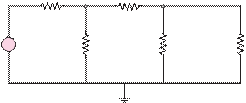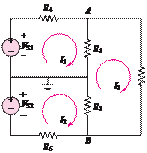### Create an Account

Already have account?

### Forgot Your Password ?

Home / Questions / Use node voltage analysis to find the voltages V1 and V2 for the circuit of Figure P31 ...

# Use node voltage analysis to find the voltages V1 and V2 for the circuit of Figure P31 32 Using node voltage analysis find the voltages V1 and V2 for the circuit of Figure

Use node voltage analysis to find the voltages V1 and

V2  for the circuit of Figure P3.1.

3.2     Using node voltage analysis, find the voltages V1 and

V2  for the circuit of Figure P3.2.

4 A                                 3 Ù

Figure P3.1

2 Ù                      1 Ù30 Ù        V110 Ù        V2

 -

20 V  +

20 Ù

30 Ù                   30 Ù

Jun 19 2020 View more View Less

#### Answer (Solved)Subscribe To Get Solution